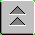Introduction to Naval Weapons Engineering

Infrared Propagation and Detection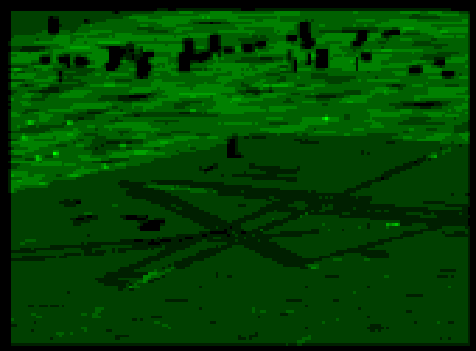In this section, we take up the problem of detecting and locating a target by the collection and processing of its thermal radiation. This is considered a passive process because the source of the radiation is the target itself. An active system, on the other hand, uses a separate source and detects the energy which is reflected off the target. For example, radar is an active system. Passive systems cannot measure range directly, because there is no means of determining the time delay between emission and reception like in radar systems, for example. However, passive systems do have the great advantage of being covert, meaning they do not necessarily give away their location in the process of detecting a target.

Electro-optical detecting systems involve the process of collecting and measuring the radiation from the target. In general, this process is called radiometry. Since we will be using many of the same principles to study how weapons systems employ electo-optics, we will adopt the standard terminology used. The following common radiometric terms and symbols will prove useful:

Flux, F. The rate of energy flow per unit time, which, of course, is power. Therefore, flux has the same units as power, namely Watts (W). The term flux may be applied either to the power leaving the target or to the power which is collected by the detector.

Exitance, M. The flux per unit area of the source, which for our purposes is also called the target. Exitance has units of W/m2. For thermal radiators, exitance is given by the Stefan-Boltzmann law, namely

M = esT4.

Incidance, E. The flux per unit area at the detector. Incidance, like exitance, has units of W/m2.

Fundamental to the process of detection is the relationship between the incidence (E) and the exitance (M). Ultimately, to quantify the detection ability of a receiver, we must derive a transfer equation, which relates the two quantities E and M. As this point the relationship is unknown, but to illustrate what we want, we may write an expression:

E = (?) M,

where (?) represents some unknown (at this time) transfer function, the parameters of which are yet to be determined. As you may guess, it certainly will depend on the area of the target and detector as well as the range between them. To proceed further, we now must make some simplifying assumptions.

The thermal radiation that is leaving the surface of the target is distributed in all directions. Depending on the shape of the object, some directions may have more or less radiation than others. In the general case, it is not possible to know the complete distribution of radiation as a function of direction without detailed knowledge of the surface of the target. However, there are two idealized shapes that can be used to make predictions.

Point Source

If there is no preferred direction, the thermal radiation will be evenly distributed in all directions. In other words, there is complete spherical symmetry. For example, if the target were a ball, it would radiate equally in all directions. In this case, it is as if the radiation where coming from a point at the center of the object.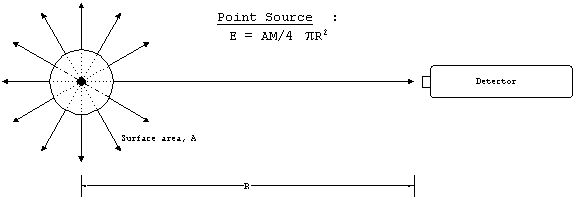Figure 1. Point source geometry.

The transfer relation has a factor of 1/4pR2 which accounts for the spreading of energy in all directions, and a factor which is the surface area of the source, A. The point source model applies well to long range detection problems, where the object appears small in the field-of-view of the detector. At shorter ranges, where the source appears as an extended object, we must use a different model.

Lambertian Source

When the source is large and/or close to the detector it will appear as an extended object, and different areas on the surface will come into the detector at different angles of incidence. To the detector, the object will appear as a two-dimensional object (i.e. flat), with an area equal to the projection of the surface area in the direction of the detector. In order to not have to worry about the exact three-dimensional shape of these objects, the source is therefore modeled as a flat plate, perpendicular to the detector, with the area of the plate equal to the projected area of the object in that direction. Furthermore, we assume that the radiation is emitted equally in all directions which leads to a slight modification of one of the numerical factors in the transfer relation. An object which can be modeled in this manner is known as a Lambertian source. The defining characteristic of a Lambertian source, is that all areas on the surface radiate equally brightly regardless of where on the surface they are. For example, the sun appears as a bright disk, with equal brightness all areas on its surface.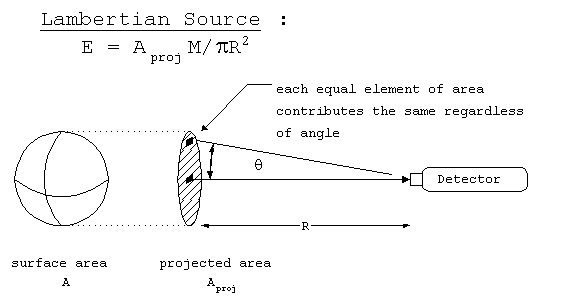Figure 2. Lambertian source
geometry.

The transfer relation for a Lambertian source uses the projected area of the source, Aproj, and is missing the factor of 4 as compared to a point source. We may compare the two formulations for a perfectly spherical source, which should yield the same results. For a sphere of radius a, the surface area A = 4pa2. The projected area Aproj = pa2. Hence, when these terms are used in the respective equations the factor of four is recovered and they are identical. Note that this will not be true for other shapes, like a cube, where the projected area is 1/6 of the total surface area, for example.

So far we have established two transfer equations, one for a point source and the other for an extended source. They are summarized below:

E = (A/4pR2)M, for a point source

and

E = (Aproj /pR2) M, for an extended (Lambertian) source.

Absorption/scattering

In the previous discussion, the transfer relation was derived on the basis of purely geometrical concepts. However, it is not true that all of the radiated energy can be captured by detectors. The intervening medium between the source and the detector, namely the atmosphere, will scatter and possibly absorb some of the energy passing through it. The overall loss in the flux is called attenuation, and is determined a variety of factors. Primarily the attenuation is a function of the wavelength and range. Additionally, it will depend on the nature of the atmosphere, such as the humidity, visibility, weather and so forth, but these factors are too variable to treat to other than empirically.

Dependence of Attenuation on Wavelength

The atmosphere is made up of a mixture of gases, predominately nitrogen and oxygen. Here is the typical composition of dry air:

 Constituent Volume % Nitrogen, N2 78 Oxygen, O2 20.9 Argon, Ar .9 Carbon dioxide, CO2 .04 (variable) Water vapor, H2O 0-2 (variable)

Table 1. Atmospheric constituents.

As in the case of selective radiators, these molecules can only absorb energy at certain wavelengths. Although nitrogen and oxygen are by far the most abundant, they do not absorb strongly in the infrared spectrum. Only water vapor and carbon dioxide play a significant role. They appear in varying amounts and therefore one can expect a variation in the amount of attenuation. Of particular note is the strong absorption by water vapor for wavelengths in the 6-8 mm band. The corresponding characteristic temperatures (i.e. the temperature which gives has these values for the peak wavelengths) are 90-210 0C. This makes detection of sources within this temperature band difficult.

Dependence of Attenuation on Range (Bouguer's Law)

The amount of attenuation by the intervening atmosphere will depend on the amount of flux. In general, the higher the flux, the greater the losses. This is similar to radioactive decay, where the decay rate is proportional to the amount of material present. In both cases, the differential equation may be solved and results in an exponential loss law. For electro-optics, this is known as Bouguer's law which states that the fraction of flux that is remains after attenuation, t, is a function of range, R:

t = e-bR

where b is the extinction coefficient, and has units of m-1. The factor t is known as the transmission coefficient, and is dimensionless. As short range, t will be close to 1.0 but will decrease and the range increases. At long range, t will be nearly zero.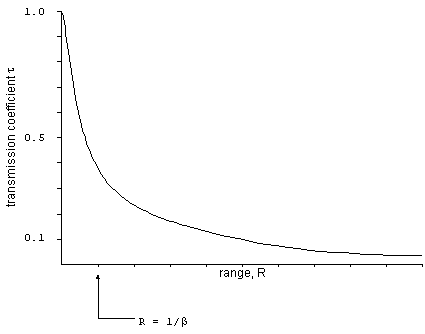Figure 3. Atmospheric attenuation.

The extinction coefficient determines the range at which the transmitted flux is significantly reduced. When the range is 1/b, the transmission coefficient will be 0.37. The extinction coefficient will generally be different for each detector, since the band of wavelengths each detector looks at are usually different. Furthermore, it will depend on the exact atmospheric conditions, including weather, season, time of day, and altitude. Here some typical values:

 Band (mm) Extinction coefficient, b 3-5 6.7 x 10-5 m-1 8-12 2.0 x 10-4 m-1

Table 2. Extinction coefficients.

The transmission coefficient, t, can be used directly in the transfer relation. For example, for a point source, the incidance is (note that t is a function of range):

E = (tA/4pR2)M.

The Collected Flux

Aperture

The incidance, E, represents the power per unit area that arrives at the detector. As a rough estimate, one could multiply the incidance by the size of the opening to the detector to determine the amount of flux that gets collected by the detector. However, this process is not 100% efficient. Some of the flux may be reflected, scattered or absorbed by the materials. Therefore, in complete analogy with our discussion of radar, we can define the effective area for collecting the incidance, which is also called the detection aperture, given the symbol Ae. The aperture is defined by:

Ae = rA

where:
r = the efficiency of the collection for the detecting system, and
A = the physical size of the opening into the detector.

The physical opening into the detector can be limited by an aperture stop (A.S.), which is physical barrier at the entrance of the detector. The aperture stop will limit the amount of flux that can enter the detector, but in turn can improve the depth of focus (which will be discussed in the next chapter).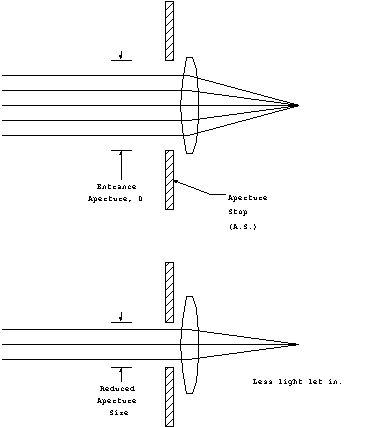Figure 4. How an aperture stop
affects the collected flux.

For a given aperture, Ae, the amount of flux that will be collected by the receiver is the product of the incidance and the aperture:

Fcollected = Ae E

Bandwidth Factor, F

Thermal radiators emit their energy over a wide band of wavelengths. In general it is not possible to design a detector which will be equally sensitive over all the wavelengths in this range. Therefore, there will be some upper and lower bounds on the wavelengths that can be detected. Designate these bounds as lupper and llower. For our purposes, we assume that the detector can pick up any energy that is within this range, and nothing that is outside of it. Since the spectrum of the radiation from a thermal emitter will not all be within the detection band, we define the bandwidth factor, F, to be the fraction of the radiation that is within the detector band.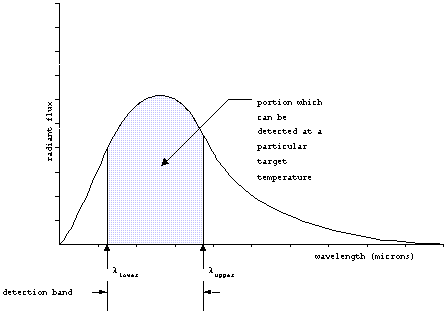Figure 5. Detection band.

The detector bandwidth factor, F, could be obtained by integrating the flux vs. wavelength curve for the source between the limits of wavelengths for the detector. This curve varies with target temperature, so it is useful to remove this dependence. The universal black-body curve represents the fraction of the total flux which is outside of the band from zero to the new variable lT, in units of mm-K.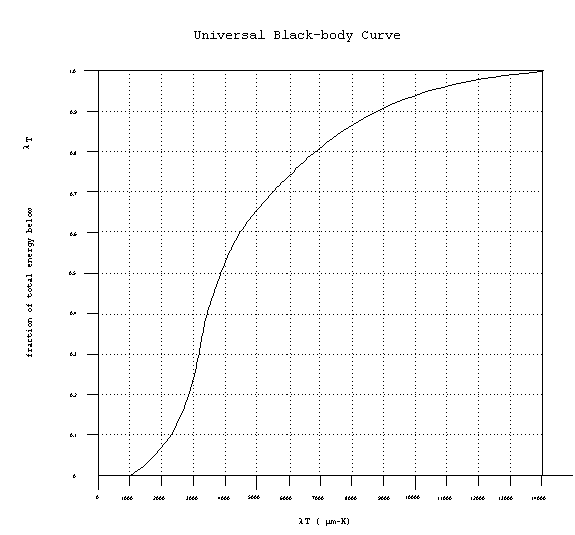Figure 6. Universal black-body
curve.

Since the variable lT now carries the temperature dependence this curve can be used for all sources. The bandwidth factor F can be found by using the curve twice: once for the lower limit and once for the upper limit, the difference being the desired factor F. This process is best illustrated by the following example.

Example: find the bandwidth factor for a detector operating between 2 and 6 microns, for a target at 500 K.

First, find the fraction of flux outside of the band from 0 to 1000 mm-K (corresponding to the smallest wavelength detectable, 2mm times the temperature, 500 K),

fraction below 0-1000 mm-K: 0.0 (i.e. nothing is detected)
Secondly, find the fraction of the flux below the band from 0 to 3000 mm-K (corresponding to the largest wavelength detectable).

fraction below of 0-3000 mm-K: 0.25

Therefore, the fraction of the total flux within the band of 1000 to 3000 mm-K is 0.25 - 0.00 = 0.25, or 25%

F = 0.25

Given the bandwidth factor, F, the flux collected and dtectable by the receiver is now:

Maximum Range Equation

Having discussed all the preliminaries, we can now put it all together and predict the maximum range at which infrared detection can take place. Similarly to radar, let Smin represent the minimum flux that the receiver can detect. Then the detection criterion can be written as

Freceiver > Smin, for successful detection.

Since the amount of flux at the receiver decreases with range, we can define the detection criterion at maximum range, where Freceiver = Smin, using the previously derived expressions:

Freceiver = AT M F Ae/(4p R2max) = Smin

Which can be solved for Rmax. Finally we substitute in the Stefan-Boltzmann law for exitance M and obtain: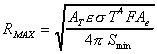Equation 1. Maximum IR detection
range in vacuum.

Note that the factor for atmospheric attenuation, t, has been omitted from this equation. Recall that the atmospheric attenuation is a function of range. This would make it impossible to write an equation for range in a closed-form. Therefore, the maximum range that is predicted by this formula, does not include atmospheric attenuation. This factor can be included as a correction, using numerical or graphical means to solve for the true range.

If the predominant source of noise is the background environment, the detector is said to background-limited. In this case Smin will depend on the temperature of the target in relation to the background. Not only will a higher temperature increase the contrast between the target and the background, but will also serve to separate their spectra as the peak wavelength shortens.

If the predominant source of noise is the detector itself, the detector is said to be noise-limited. In this, the minimum flux can be written as

Smin = (S/N) NEP

where:
S/N = the signal-to-noise ratio required for detection, and
NEP = the noise-equivalent power.

Example: find the maximum detection range for a point-source target, with surface area 2 m2, emissivity of 0.95 and temperature of 500 K, given the following detector characteristics:

Aperture = 0.1 m2

Detection band = 2-6 mm

Smin = 6 x 10-7 W

Step 1: Compute F, using the universal black-body curve with arguments of 1000 and 6000 mm-K F = 0.75

Step 2: Calculate range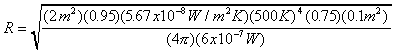R = 8190 m